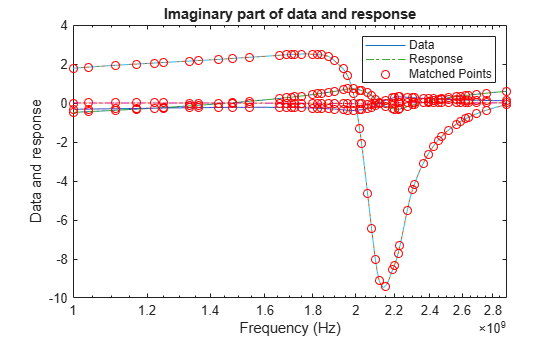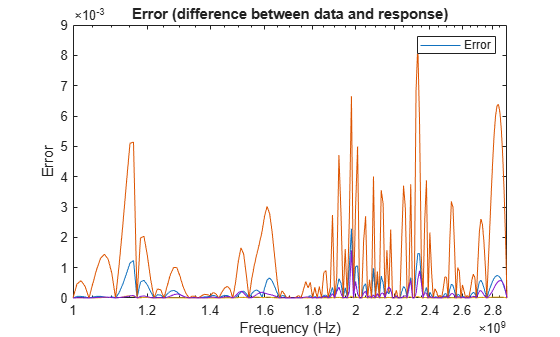# zpk

Compute zeros, poles, and gain of rational object

Since R2020a

## Syntax

``[z,p,k,dcgain] = zpk(fit)``

## Description

example

````[z,p,k,dcgain] = zpk(fit)` returns the zeros, poles, gain, and DC gain of a rational object.```

## Examples

collapse all

Create an S-Parameters object from the file named `default.s2p`. Perform rational fitting of the S-Parameters.

```S = sparameters('default.s2p'); fit = rational(S,Display='plot')``````fit = rational with properties: NumPorts: 2 NumPoles: 52 Poles: [52x1 double] Residues: [2x2x52 double] DirectTerm: [2x2 double] ErrDB: -22.6872 ```

Calculate the zeros, poles, gain, and DC gain of the rational object.

`[z,p,k,dcgain] = zpk(fit)`
```z=2×2 cell array {51x1 double} {51x1 double} {51x1 double} {51x1 double} ```
```p=2×2 cell array {52x1 double} {52x1 double} {52x1 double} {52x1 double} ```
```k = 2×2 1010 × 1.0544 -0.0194 0.9158 0.0377 ```
```dcgain = 2×2 0.1289 -0.0838 -0.1209 0.7649 ```

## Input Arguments

collapse all

Rational fit, specified as a `rational` or `rfmodel.rational` object.

## Output Arguments

collapse all

Zeroes of the fit, returned as a 1-D array of doubles or a 3-D array of doubles.

Poles of the fit, returned as a 1-D array of doubles or a 3-D array of doubles.

Gain of the fit, returned as a 2-D array of doubles. `k` is the coefficient of the rational function when poles and zeros are expressed as monic polynomials in `S`.

DC gain of the fit, returned as 2-D array of doubles for zero frequency response.

## Version History

Introduced in R2020a